{{announcement.body}}
{{announcement.title}}

# My Newbie Challenges With Matplotlib

DZone 's Guide to

# My Newbie Challenges With Matplotlib

### This great tutorial of Matplotlib is perfect for developers new to Python and NumPy, covering a few challenges and solutions with the library.

· Big Data Zone ·
Free Resource

Comment (0)

Save
{{ articles.views | formatCount}} Views

In this article, I would like to share the challenges I faced (and the solutions!) as a Python newbie using Matplotlib in anger for the first time…

Recently I was tasked with developing a Python-based workflow as well as an article for my employer. The workflow involved generating some Technical Analysis related charts and the article involved plotting some Volatility Surfaces and various Curves.

As someone quite new to Python and never really used Matplotlib previously, this presented quite a challenge.

Sure I had read a few articles/tutorials on Matplotlib — but quite often these were very ‘atomic’ — each focusing on individual bits of functionality but not how you could combine multiple features into one presentable chart. Or they used different approaches/interfaces to achieve a similar end objective — making it harder to combine the snips of code. And none of these covered all the different features I wanted to apply — so I had to glean hints and guidance from multiple sources — just to make one chart look pretty.

Each chart I had to create presented a slightly different challenge — which meant more googling and more testing out snippets. So, I promised myself to document all the challenges and solutions to help others in a similar situation — and this article is the result of that promise. I hope you find it interesting as well as useful….

## Aesthetics First

As you can imagine, most of my challenges were around the aesthetics of my charts. For my Technical Analysis workflow, I needed to replicate some charts that are available in my employer’s desktop application and they look much better than the ‘black axes on white background’ stuff that Matplotlib generates by default.

The desktop application generates some very contemporary looking charts with black/charcoal backgrounds and contrasting colour line plots: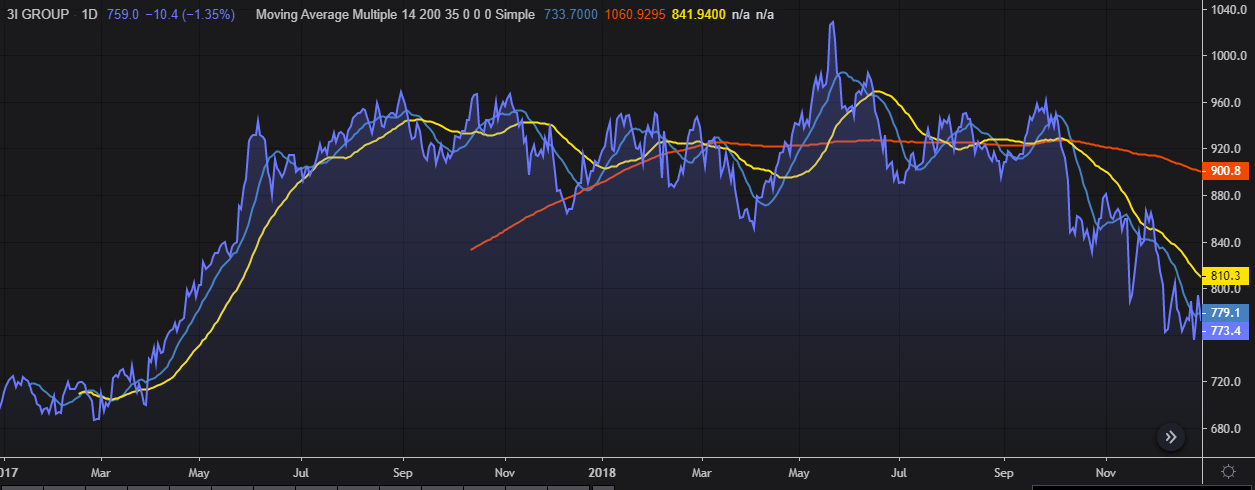Now, clearly, I was not going to be able to replicate the hours of work and polish that had gone into those — but I did want to replicate the look to some degree at least. So, the first challenge was to make the default Matplotlib stuff look something like the above:

0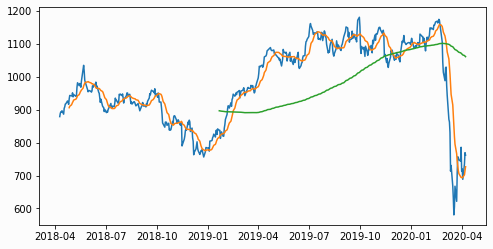The above was generated using the following code:
Python

`xxxxxxxxxx`
1

1
`x = close.index`
2
`plt.plot(x,close, label='Close')`
3
`plt.plot(x,sma14,label="SMA 14")`
4
`plt.plot(x,sma200,label="SMA 200")`
5
`plt.show()`

Where close is my Closing Price data and sma14 and sma200 represent my short and long period Moving Averages data.

A few immediately obvious things that needed fixing:

• Change the background and foreground colours
• Make the chart bigger
• Label my line plots
• Title the chart

I assumed I would be able to call something like the following to change the background colour — but it seems it is not that simple.

Python

`xxxxxxxxxx`
1

1
`plt.set_facecolor('black')`

Instead, I had to do set the figure and axes colours individually (where ‘0.25’ is a grey shade I quite liked):

Python

`xxxxxxxxxx`
1

1
`plt.rcParams["figure.facecolor"] = '0.25'`
2
`plt.rcParams["axes.facecolor"] = '0.25'`

I then found that I needed to access the figure and the axes individually using the subplots call (in order to change the colour of tick markers). In doing so I discovered I could roll the figsize and facecolor into the same subplots access call - saving a couple of lines of code:

Python

`xxxxxxxxxx`
1

1
`x = close.index`
2
`fig, ax = plt.subplots(facecolor='0.25',figsize=(28,8))`
3
`ax.set_facecolor('0.25')ax.plot(x,close, label='Close',color='y')`
4
`ax.plot(x,sma14,label="SMA 14", color='g')`
5
`ax.plot(x,sma200,label="SMA 200",color='tab:purple')ax.tick_params(axis='both', colors='w', labelsize=20)`
6
`plt.ylabel('Close',color='y')`
7
`plt.legend(loc='upper center',fontsize='x-large')`
8
`plt.title(f"Simple Moving Average : {ric}",color='w')`
9
`plt.show()`

The plt.subplots() call returns the enclosing figure (container) and the axes so I can interact with each individually — and whilst doing so I was able to set the size and colour of the figure.

In addition, I did the following

• call ax.set_facecolor() to set the axes (plot area) colour
• colour each line plot by adding the ‘color’ parameter where y=yellow, g=green and ‘tab:purple’ (which is from the Tableau palette)
• ax.tick_params() to change the colour of the tick values on both axes from the default black to a more legible white, and make the font a bit bigger
• plt.legend() to show the actual line plot labels in a nice little panel
• plt.title() for the chart title

All that brought me much closer to the prettier chart I was looking for:

0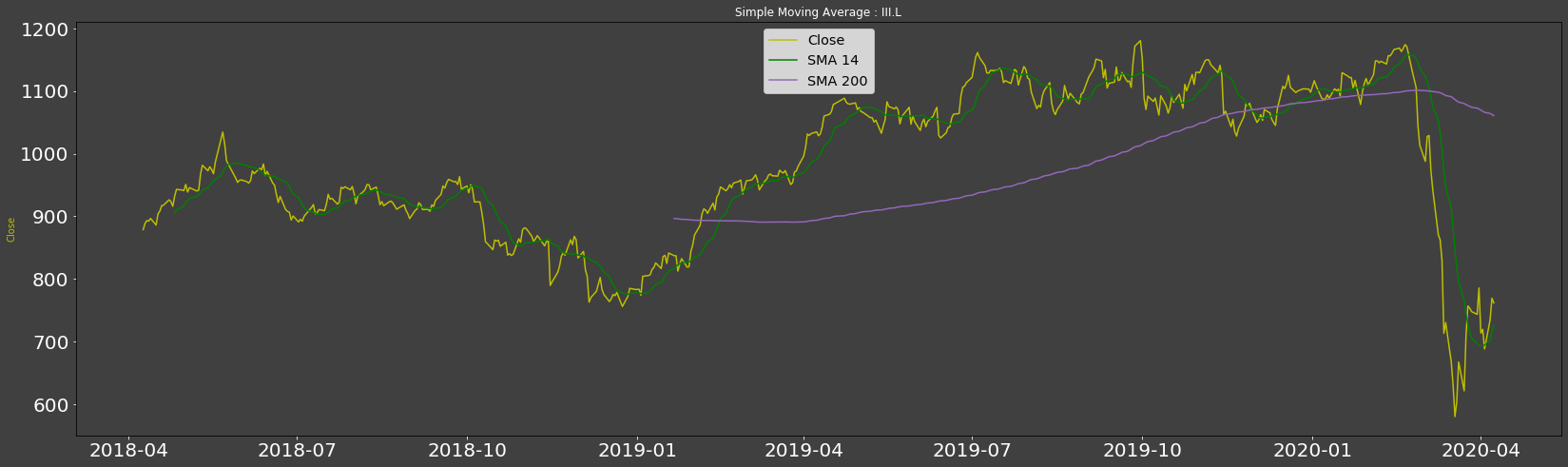At this point, I had been testing with a daily data interval and was thinking things were looking pretty good. I then tested with an hourly data interval and was not impressed with what I saw: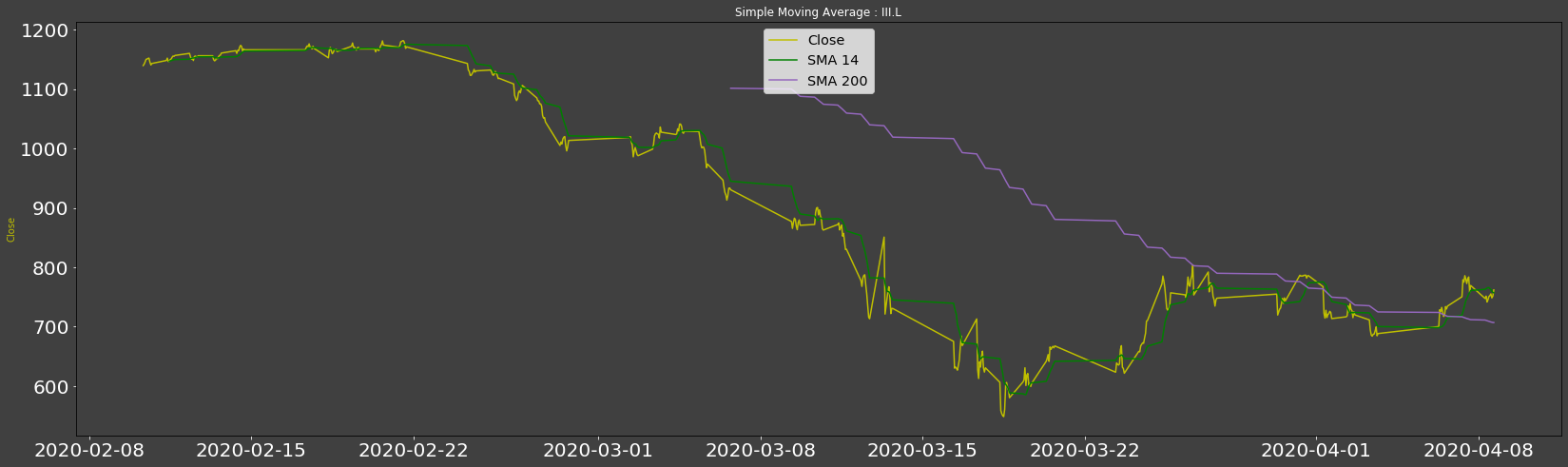Much googling later I worked out that I could use the numpy.arange function to produce some evenly spaced data — which worked well, but my x-axis no longer showed the date. So I added the following custom Formatter to restore the dates to the x-axis.

Python

`xxxxxxxxxx`
1

1
`class MyFormatter(Formatter):`
2
`    def __init__(self, dates, fmt='%Y-%m-%d'):`
3
`        self.dates = dates`
4
`        self.fmt = fmtdef __call__(self, x, pos=0):`
5
`        'return date x at position pos'`
6
`        ind = int(round(x))`
7
`        if ind>=len(self.dates) or ind<0: return ''`
8
`        return self.dates[ind].strftime(self.fmt)`

I then reference MyFormatter as part of my final plot code as follows:

Python

`xxxxxxxxxx`
1
14

1
`x = close.index`
2
`fig, ax = plt.subplots(facecolor='0.25',figsize=(28,8))`
3
`ax.set_facecolor('0.25')`
4
`formatter = MyFormatter(x)`
5
`ax.xaxis.set_major_formatter(formatter)`
6
`ax.plot(np.arange(len(x)),close, label='Close',color='y')`
7
`ax.plot(np.arange(len(x)),sma14,label="SMA 14", color='g')`
8
`ax.plot(np.arange(len(x)),sma200,label="SMA 200", color='tab:purple')`
9
`ax.tick_params(axis='both', colors='w', labelsize=20)`
10
`fig.autofmt_xdate()`
11
`plt.ylabel('Close',color='y')`
12
`plt.legend(loc='upper center',fontsize='x-large')`
13
`plt.title(f"Simple Moving Average : {ric}",color='w')`
14
`plt.show()`
8
`ax.plot(np.arange(len(x)),sma200,label="SMA 200", color='tab:purple')`

I also added the fig.autofmat_xdate() to rotate the x-axis labels and avoid overlap. The final result is a much smoother plot with evenly spaced date labels: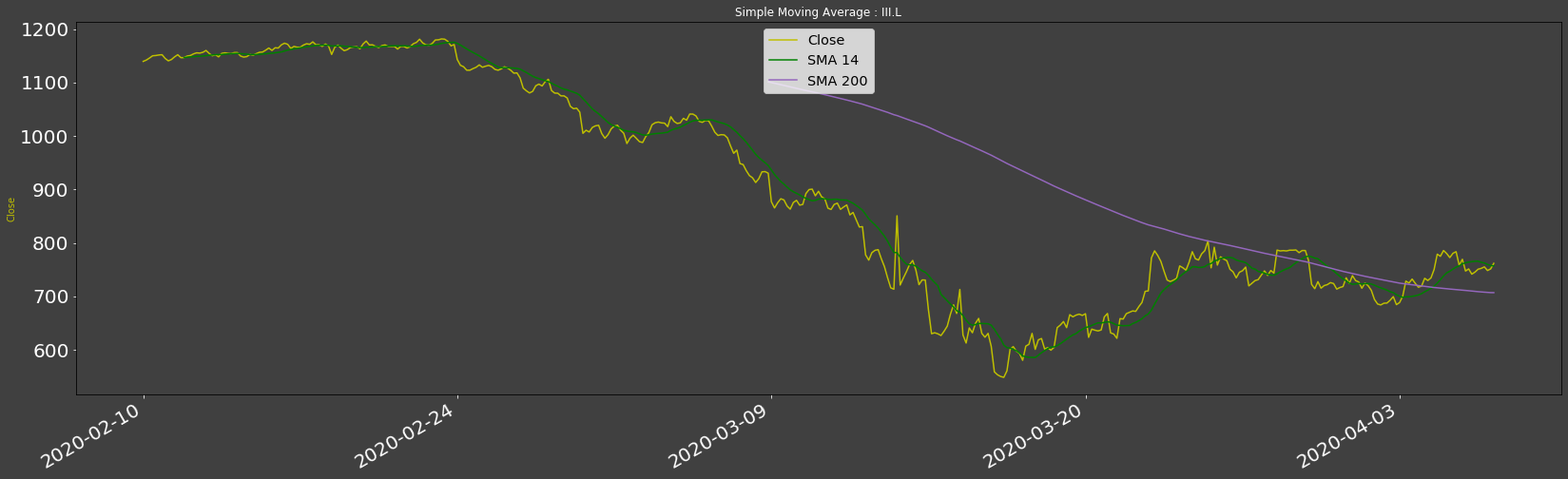## Stacked Charts

For the Simple Moving averages chart, it made sense to show the Closing price line on the same plot as the short and long Moving Average lines — to contrast the Moving Averages with the Close price.

However, for my next two charts, this would not be ideal — I really need to show the lines as separate plots — albeit sharing the same x-axis. In other words, I wanted to ‘stack’ the two charts on top of each other.

First, I tried to create two charts and position them on top of each other — but the gap between the charts was too large and the x-axis was repeated unnecessarily.

I then came across the GridSpec class which allows the user to ‘Specify the geometry of the grid where a subplot will be placed ‘— sounded perfect!

Using GridSpec I created a grid of 2 rows and a single column and then added a subplot to each row. I then plotted my Closing Price chart in the first row and my other chart in the 2nd one:

Python

`xxxxxxxxxx`
1

1
`# Create a 2(rows) x 1(col) Grid`
2
`gs1 = gridspec.GridSpec(2, 1)# Closing price chart at row 0`
3
`axc = fig.add_subplot(gs1)`
4
`axc.plot(np.arange(len(close.index)), close)# RSI chart at row 1`
5
`axr = fig.add_subplot(gs1)`
6
`axr.plot(np.arange(len(rsi.index)), rsi.values,color='b')# minimise the gap between the rows`
7
`gs1.update(wspace=0.025, hspace=0.0)plt.show()`

Once I added in the additional stuff for labels, tick params, axis formatting etc I ended up with the following: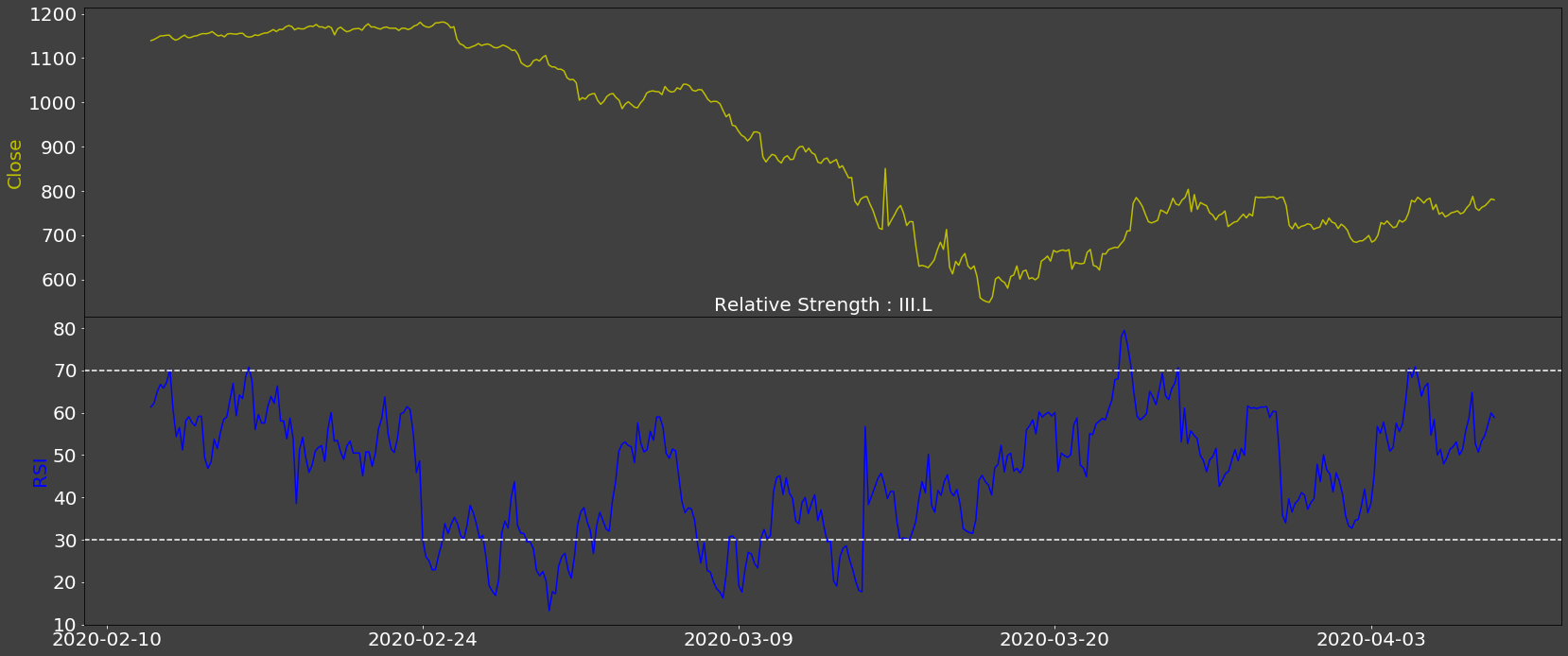Note the dashed ‘RSI threshold’ lines at 70 and 30 — which I added with the following code:

Python

`xxxxxxxxxx`
1

1
`plt.axhline(y=70, color='w',linestyle='--')`
2
`plt.axhline(y=30, color='w',linestyle='--')`

I then re-used all the above techniques to create a Stochastic chart that you can see below:

0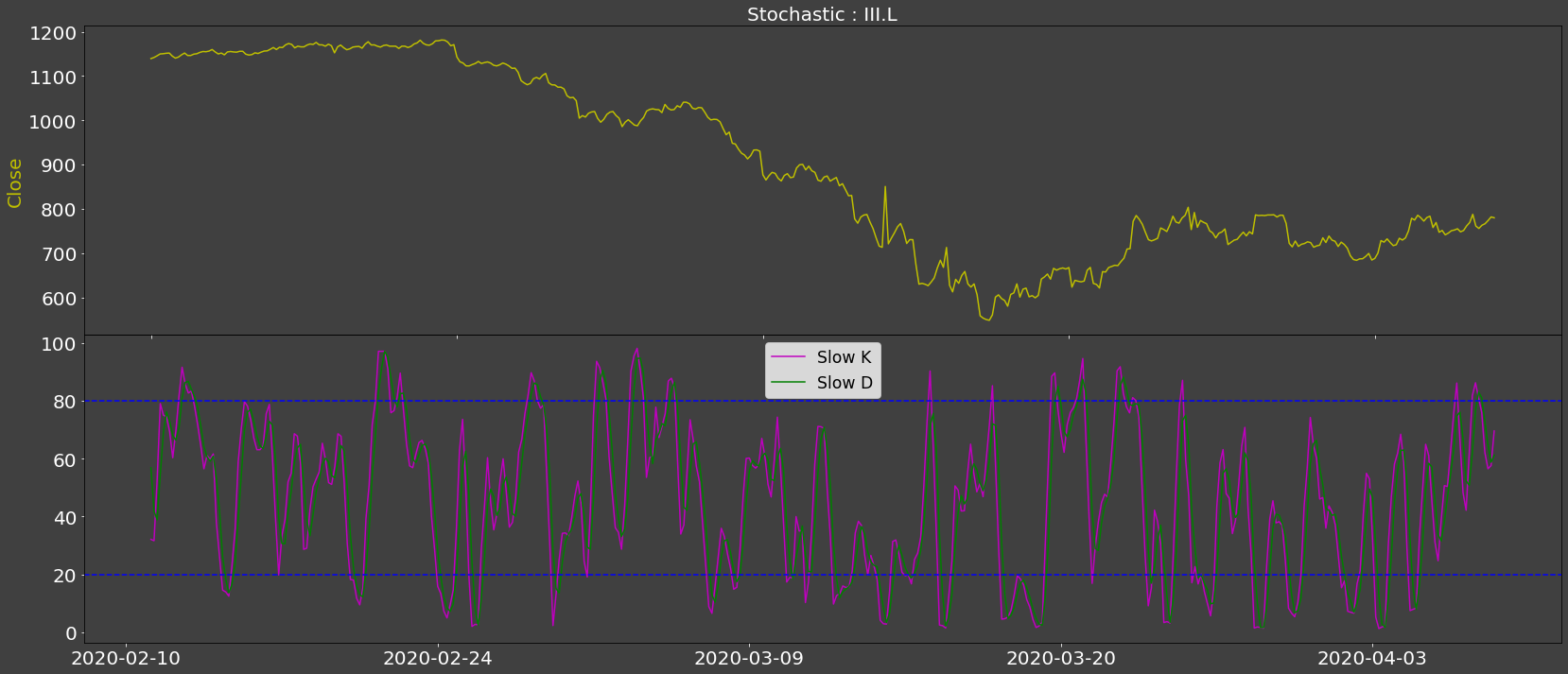I have not included the full code for the above charts here to keep the article as short as possible, so I will provide a link to the full source at the end.

## Volatility Surfaces and Curves

My 2nd piece of work required me to include some Volatility Surfaces and a few Curves in the article I was writing. Somebody else has already created some basic plots of the types I needed — however, I did not want the default Matplotlib look: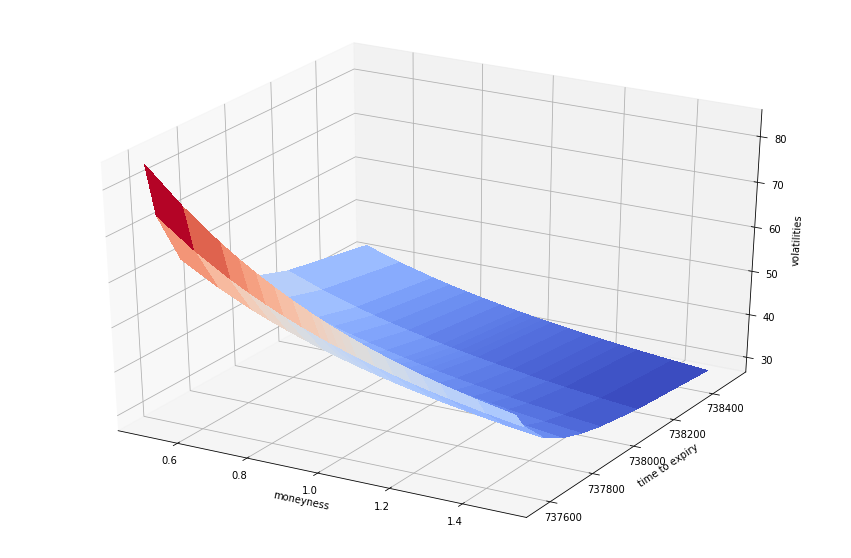I didn’t like the colours, the position of the axes labels nor the Y-axis tick labels. I already knew how to do the colours which just left the tick labels and axes label positioning.

So, why were the Y-axis of ‘time to expiry’ ticks labelled with numeric values? Because, for this plot type, the Matplotlib library requires the date to be expressed in ‘number of days since UTC’ — e.g. 737600 = 25 June 2020.

I initially tried to find a way of passing in the date in two formats, one for the tick labels and one for the plot — when calling the plot function. However, I soon realised I could just use a custom Formatter again:

Python

`xxxxxxxxxx`
1

1
`# Convert float date back to Y-m-d for the y axis tick labels`
2
`def format_date(x, pos=None):`
3
`    import matplotlib.dates as dates    return dates.num2date(x).strftime('%Y-%m-%d') `

And the axes label spacing/position could be improved via the labelpad attribute. So, the following code snippet:

Python

`xxxxxxxxxx`
1

1
`ax = plt.axes(projection='3d')`
2
`ax.set_facecolor('0.25')`
3
`ax.set_title('Vol Surface for : VW',color='w')`
4
`ax.set_xlabel('Moneyness',color='y',labelpad=10)`
5
`ax.set_ylabel('Expiry',color='y',labelpad=15)`
6
`ax.set_zlabel('Volatilities',color='y')`
7
`ax.tick_params(axis='both', colors='w')ax.w_yaxis.set_major_formatter(ticker.FuncFormatter(format_date))`
8
`       `
9
`surf = ax.plot_surface(X,Y,Z, cmap=cm.coolwarm, linewidth=0, antialiased=False)plt.show()`

produces the following — which I hope you will agree, looks more appealing: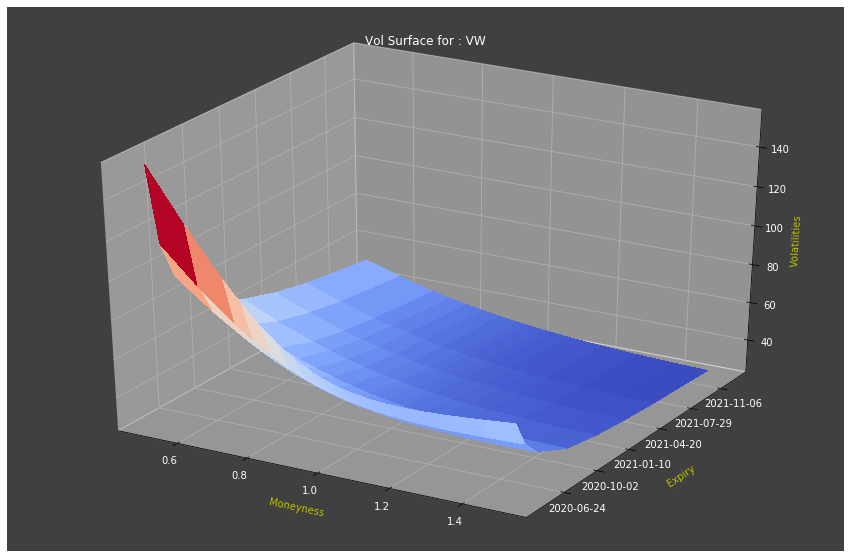## A Bit of a Cheat!

This final one, I have to admit is a bit of a cheat— there is probably a correct way of doing it — but my ‘fix’ worked!

I had to plot a chart with data for three instruments over a given period. Should be simple enough — but for some reason, the chart looked like this: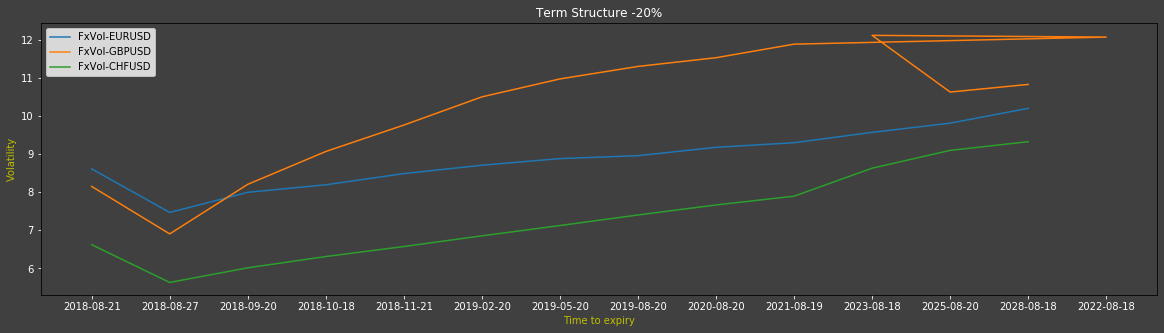After a bit of digging, it turned out that the GBP data had an extra data point for 2022 (which the EUR and CHF data did not). However, because I was plotting the EUR data before the GBP data, Matplotlib created the mess you see above.

So, how did I ‘fix’ this? I just plotted the GBP data first — giving me the following neater output: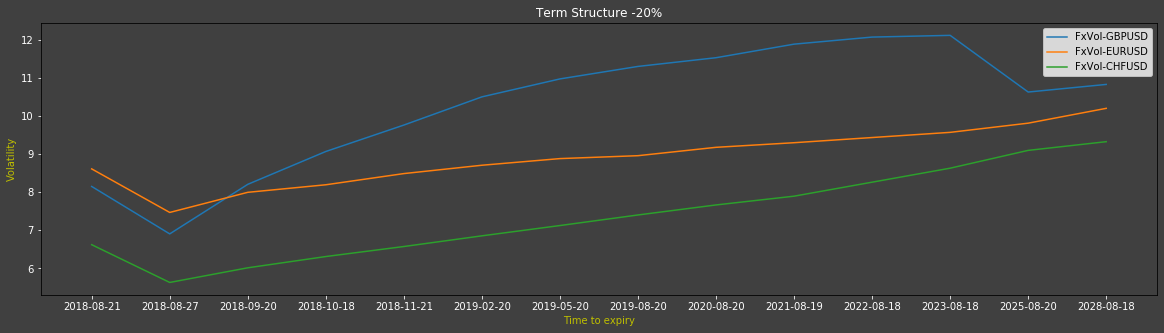The takeaway from this is that the sequence of a multi-line plot does seem to matter when dealing with non-identical x-axis values. I suppose that if each of the currencies had different dates, I would have to pad/fill the dates out somehow.

## Closing Words

I did have several other issues around creating and formatting these charts — but these were more to do with the data format not being quite how I needed/wanted it to be — rather than Matplotlib issues.

So, thanks for reading and I hope you found it interesting if not entirely useful!

Full Source code:
As promised, here are the links for the full source code behind the snippets I used above. You will need various licences/accounts to run them — however, I am hopeful that you can lift the relevant charting snippets and re-use them with your data in your Python code.

Volatility Surfaces and Curves on GitHub

Technical Analysis Workflow on GitHub

Topics:
market data, matplotlib, python, technical analysis chart software, volatility

Comment (0)

Save
{{ articles.views | formatCount}} Views

Opinions expressed by DZone contributors are their own.